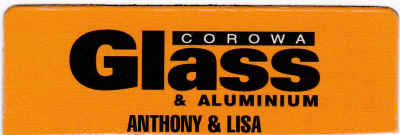## WAHGUNYAH SPEEDWAY TRACK CHAMPIONSHIP STANDINGS# Points  StructureHeats
1st = 60
2nd = 57
3rd = 54
4th = 51
5th = 48
6th = 45
7th = 42
8th = 39
9th = 36
10th = 33
11th = 30
12th = 27
13th = 24
14th = 21

Final
1st = 120
2nd = 114
3rd = 108
4th = 102
5th = 96
6th = 90
7th = 84
8th = 78
9th = 72
10th = 66
11th = 60
12th = 54
13th = 48
14th = 42
15th = 36
16th = 30
17th = 24
18th = 18
19th = 12
20th = 6